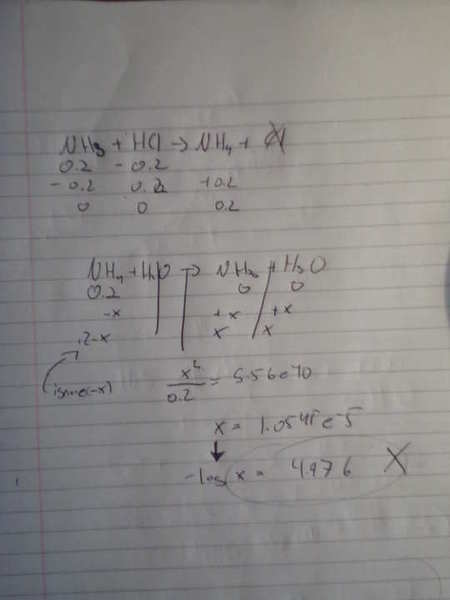# Titration: Calculate pH at Equivalence Point (NH3 & HCl)

• woox

## Homework Statement

Calculate the pH at the equivalence point for the titration of 0.20 M NH3 with 0.20 M HCl? Kb of ammonia is 1.8e-5

This is just a old test I am going over, I managed to get a pH of 4.98. but that is not right the right answer is 5.12 and I can't figure out why. I would assume you could use the henderson equation but I only get 4.98 which is wrong.

Any help?

acid base titration equivalence point calculation

Note: you should not use Henderson-Hasselbalch, however, you should not forget about dilution.

Even if I did not use HH equation I am getting the wrong answer.

So show details of your work, hard to say what you did wrong not knowing what you did.

So show details of your work, hard to say what you did wrong not knowing what you did.
k... using ICE table below: